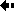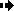## Multi-locus population genetics - How does the amount of linkage disequilibrium change?Population geneticists try to calculate the change in linkage disequilibrium over generations. In the absence of selection, it is done like this:

In the absence of selection, the frequencies of each gene will be constant, but the frequencies of the haplotypes can be altered by recombination.

Again, take the case of two loci with two alleles each. Let the gene frequency of A1 be p1, A2 be p2, B1 be q1 and B2 be q2.

haplotype frequency in population

A1B1 a = p1q1 + DA1B2 b = p1q2 - DA2B1 c = p2q1 - DA2B2 d = p2q2 + D

The frequency can only be altered by recombination in the double heterozygotes A1B1/A2B2 and A1B2/A2B1. When recombination takes place in an A1B1/A2B2 individual, the number of A1B1 haplotypes is decreased; when it takes place in an A1B2/A2B1 individual, the number of A1B1 is increased. To be exact, half the genes of an A1B1/A2B2 double heterozygote are A1B1; when recombination hits between the loci the frequency of A1B1 decreases by an amount -1/2. Similarly, recombination in an A1B2/A2B1 individual increases the frequency of A1B1 by an amount +1/2.

The frequency of A1B1/A2B2 heterozygotes in the population is 2ad and of A1B2/A2B1 is 2bc. The frequency of recombination between the two loci is defined as r.

a +1 generation = a - r (ad - bc ).

Now, the expression (ad - bc ) is simply equal to the linkage disequilibrium D.

If D = 0, i.e. if the genes are randomly associated, the haplotype frequencies are constant: a + 1 generation = a.

But if there is an excess of A1B1 haplotypes, the excess decreases by an amount rD per generation. The same relation holds true for any successive pair of generations. We can see what is happening graphically if we substitute for a in the equation:

a + 1 generation = p1q1 + D - rD a + 1 generation - p1q1 = (1-r )D

The difference between a and p1q1 is the amount of 'excess' of the A1B1 haplotype (i.e. the amount by which the frequency exceeds the random frequency). It is also equal to the linkage disequilibrium (D = a - p1q1). Therefore

D + 1 generation = (1-r)DPrevious Next